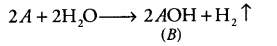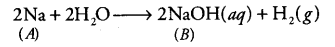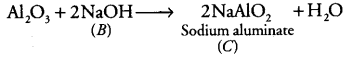# An alkali metal A gives a compound B (molecular mass = 40) on reacting with water

An alkali metal A gives a compound B (molecular mass = 40) on reacting with water. The compound B gives a soluble compound C on treatment with aluminium oxide. Identify A, B and C and give the reactions involved.

Let the atomic weight of alkali metal A be x. When it reacts with water, it forms a compound B having molecular mass 40. Let the reaction beAccording to the question,
x + 16 + 1 = 40 (Given)
x = 40-17 = 23
It is the atomic weight of Na (sodium).
Therefore, the alkali metal (A) is Na and the reaction isSo, compound B is sodium hydroxide (NaOH).
Sodium hydroxide reacts with aluminium oxide (Al2O3) to give sodium aluminate (NaAlO2). Thus, C is sodium aluminate (NaAlO2). The reaction involved is Al2O3+